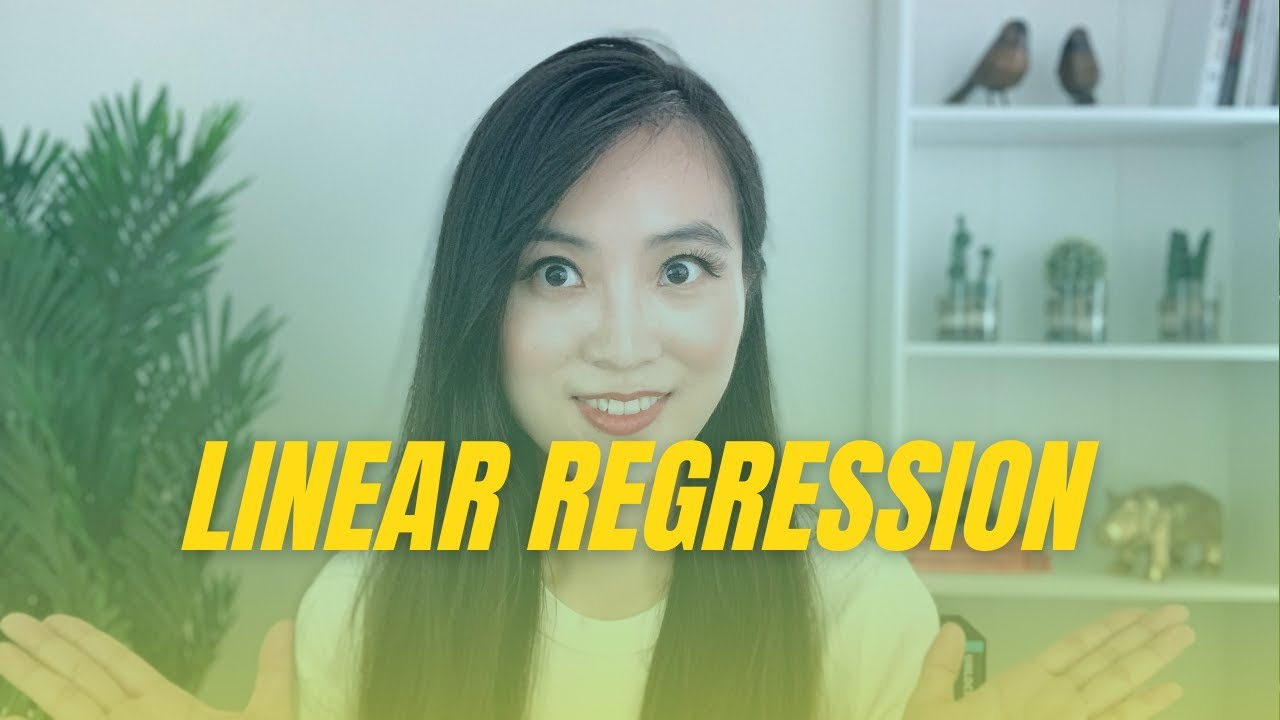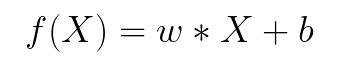1618623515

# Linear Regression in Python | Gradient Descend | Data Science Interview Machine Learning Interview

Linear Regression in Python | Gradient Descend | Data Science Interview Machine Learning Interview

TIMESTAMP

• 00:00 Linear Regression Overview
• 06:32 Linear Regression Implementation
• 10:48 Time and Space Complexity

#python #data-science #interview-questions

## Buddha Community1625843760

## Python Packages in SQL Server – Get Started with SQL Server Machine Learning Services

##### Introduction

When installing Machine Learning Services in SQL Server by default few Python Packages are installed. In this article, we will have a look on how to get those installed python package information.

##### Python Packages

When we choose Python as Machine Learning Service during installation, the following packages are installed in SQL Server,

• revoscalepy – This Microsoft Python package is used for remote compute contexts, streaming, parallel execution of rx functions for data import and transformation, modeling, visualization, and analysis.
• microsoftml – This is another Microsoft Python package which adds machine learning algorithms in Python.
• Anaconda 4.2 – Anaconda is an opensource Python package

#machine learning #sql server #executing python in sql server #machine learning using python #machine learning with sql server #ml in sql server using python #python in sql server ml #python packages #python packages for machine learning services #sql server machine learning services1603753200

## ML Optimization pt.1 - Gradient Descent with Python

So far in our journey through the Machine Learning universe, we covered several big topics. We investigated some regression algorithms, classification algorithms and algorithms that can be used for both types of problems (SVM**, **Decision Trees and Random Forest). Apart from that, we dipped our toes in unsupervised learning, saw how we can use this type of learning for clustering and learned about several clustering techniques.

We also talked about how to quantify machine learning model performance and how to improve it with regularization. In all these articles, we used Python for “from the scratch” implementations and libraries like TensorFlowPytorch and SciKit Learn. The word optimization popped out more than once in these articles, so in this and next article, we focus on optimization techniques which are an important part of the machine learning process.

In general, every machine learning algorithm is composed of three integral parts:

1. loss function.
2. Optimization criteria based on the loss function, like a cost function.
3. Optimization technique – this process leverages training data to find a solution for optimization criteria (cost function).

As you were able to see in previous articles, some algorithms were created intuitively and didn’t have optimization criteria in mind. In fact, mathematical explanations of why and how these algorithms work were done later. Some of these algorithms are Decision Trees and kNN. Other algorithms, which were developed later had this thing in mind beforehand. SVMis one example.

During the training, we change the parameters of our machine learning model to try and minimize the loss function. However, the question of how do you change those parameters arises. Also, by how much should we change them during training and when. To answer all these questions we use optimizers. They put all different parts of the machine learning algorithm together. So far we mentioned Gradient Decent as an optimization technique, but we haven’t explored it in more detail. In this article, we focus on that and we cover the grandfather of all optimization techniques and its variation. Note that these techniques are not machine learning algorithms. They are solvers of minimization problems in which the function to minimize has a gradient in most points of its domain.

### Dataset & Prerequisites

Data that we use in this article is the famous Boston Housing Dataset . This dataset is composed 14 features and contains information collected by the U.S Census Service concerning housing in the area of Boston Mass. It is a small dataset  with only 506 samples.For the purpose of this article, make sure that you have installed the following _Python _libraries:

• **NumPy **– Follow this guide if you need help with installation.
• **SciKit Learn **– Follow this guide if you need help with installation.
• Pandas – Follow this guide if you need help with installation.

Once installed make sure that you have imported all the necessary modules that are used in this tutorial.

``````import pandas as pd
import numpy as np
import matplotlib.pyplot as plt

from sklearn.model_selection import train_test_split
from sklearn.metrics import mean_squared_error
from sklearn.preprocessing import StandardScaler
from sklearn.linear_model import SGDRegressor
``````

Apart from that, it would be good to be at least familiar with the basics of linear algebracalculus and probability.

### Why do we use Optimizers?

Note that we also use simple Linear Regression in all examples. Due to the fact that we explore optimizationtechniques, we picked the easiest machine learning algorithm. You can see more details about Linear regression here. As a quick reminder the formula for linear regression goes like this:where w and b are parameters of the machine learning algorithm. The entire point of the training process is to set the correct values to the w and b, so we get the desired output from the machine learning model. This means that we are trying to make the value of our error vector as small as possible, i.e. to find a global minimum of the cost function.

One way of solving this problem is to use calculus. We could compute derivatives and then use them to find places where is an extrema of the cost function. However, the cost function is not a function of one or a few variables; it is a function of all parameters of a machine learning algorithm, so these calculations will quickly grow into a monster. That is why we use these optimizers.

#ai #machine learning #python #artificaial inteligance #artificial intelligence #batch gradient descent #data science #datascience #deep learning #from scratch #gradient descent #machine learning #machine learning optimizers #ml optimization #optimizers #scikit learn #software #software craft #software craftsmanship #software development #stochastic gradient descent1619518440

## top 30 Python Tips and Tricks for Beginners

Welcome to my Blog , In this article, you are going to learn the top 10 python tips and tricks.

### 8) Check The Memory Usage Of An Object.

#python #python hacks tricks #python learning tips #python programming tricks #python tips #python tips and tricks #python tips and tricks advanced #python tips and tricks for beginners #python tips tricks and techniques #python tutorial #tips and tricks in python #tips to learn python #top 30 python tips and tricks for beginners1610872689

## Data Science With Python Training | Python Data Science Course | Intellipaat

In this Data Science With Python Training video, you will learn everything about data science and python from basic to advance level. This python data science course video will help you learn various python concepts, AI, and lots of projects, hands-on demo, and lastly top trending data science and python interview questions. This is a must-watch video for everyone who wishes o learn data science and python to make a career in it.

#data science with python #python data science course #python data science #data science with python1617687120

## How I'd Learn Data Science If I Were To Start All Over Again

A couple of days ago I started thinking if I had to start learning machine learning and data science all over again where would I start? The funny thing was that the path that I imagined was completely different from that one that I actually did when I was starting.

I’m aware that we all learn in different ways. Some prefer videos, others are ok with just books and a lot of people need to pay for a course to feel more pressure. And that’s ok, the important thing is to learn and enjoy it.

So, talking from my own perspective and knowing how I learn better I designed this path if I had to start learning Data Science again.

As you will see, my favorite way to learn is going from simple to complex gradually. This means starting with practical examples and then move to more abstract concepts.

#data-science #machine-learning #artificial-intelligence #python-top-story #data-science-top-story #learn-python #learn-data-science